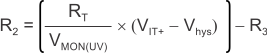ZHCS840G February   2012  – February 2019

PRODUCTION DATA.

1. 特性
2. 应用
3. 说明
1.     Device Images
4. 修订历史记录
5. Pin Configuration and Functions
6. Specifications
7. Detailed Description
1. 7.1 Overview
2. 7.2 Functional Block Diagram
3. 7.3 Feature Description
4. 7.4 Device Functional Modes
8. Application and Implementation
1. 8.1 Application Information
2. 8.2 Typical Application
1. 8.2.1 Design Requirements
2. 8.2.2 Detailed Design Procedure
3. 8.2.3 Application Curves
3. 8.3 Do's and Don'ts
9. Power-Supply Recommendations
10. 10Layout
11. 11器件和文档支持
1. 11.1 器件支持
1. 11.1.1 开发支持
2. 11.1.2 器件命名规则
2. 11.2 文档支持
3. 11.3 接收文档更新通知
4. 11.4 社区资源
5. 11.5 商标
6. 11.6 静电放电警告
7. 11.7 术语表
12. 12机械、封装和可订购信息

• DSE|6
• DDC|6

#### 8.2.2.1 Resistor Divider Selection

Use Equation 1 through Equation 4 to calculate the resistor divider values and target threshold voltages.

Equation 1. RT = R1 + R2 + R3

Select a value for RT such that the current through the divider is approximately 100 times higher than the input current at the INA+ and INB– terminals. The resistors can have high values to minimize current consumption as a result of low-input bias current without adding significant error to the resistive divider. See the application note Optimizing Resistor Dividers at a Comparator Input (SLVA450) for details on sizing input resistors.

Use Equation 2 to calculate the value of R3.

Equation 2.where

Use Equation 3 or Equation 4 to calculate the value of R2.

Equation 3.where

Equation 4.where

The worst-case tolerance can be calculated by referring to Equation 13 in application report SLVA450, Optimizing Resistor Dividers at a Comparator Input (available for download at www.ti.com). An example of the rising threshold error, VMON(OV), is given in Equation 5.

Equation 5.